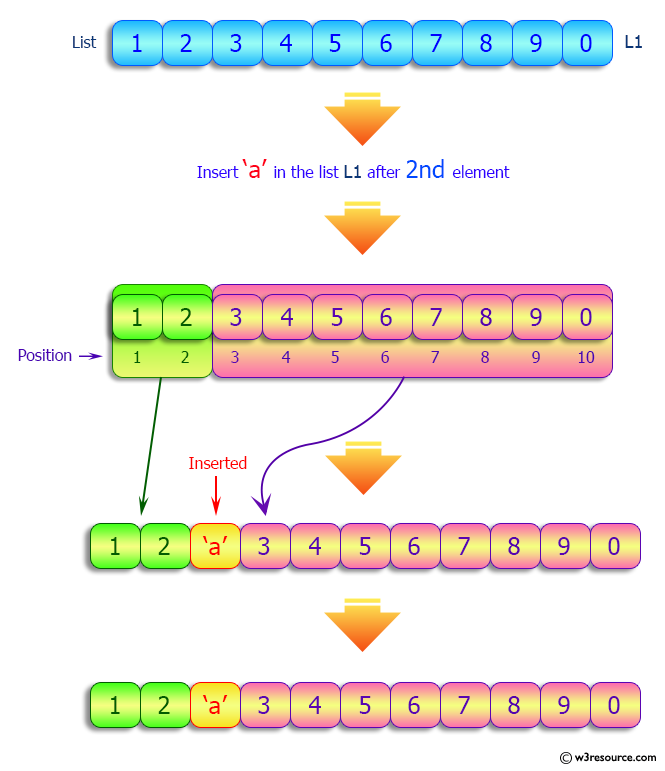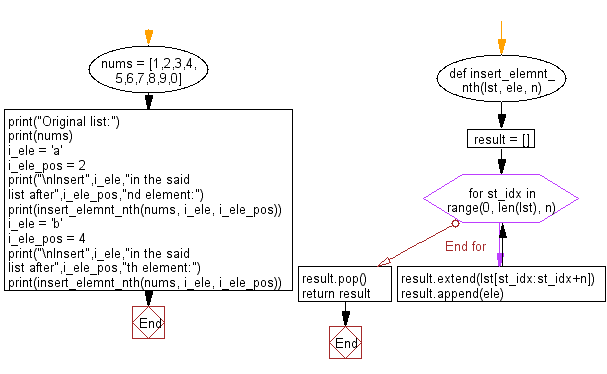﻿ Python: Insert an element in a given list after every nth position - w3resource# Python: Insert an element in a given list after every nth position

## Python List: Exercise - 170 with Solution

Write a Python program to insert an element in a given list after every nth position.

Sample Solution:

Python Code:

``````def insert_elemnt_nth(lst, ele, n):
result = []
for st_idx in range(0, len(lst), n):
result.extend(lst[st_idx:st_idx+n])
result.append(ele)
result.pop()
return result

nums = [1,2,3,4,5,6,7,8,9,0]
print("Original list:")
print(nums)
i_ele = 'a'
i_ele_pos = 2
print("\nInsert",i_ele,"in the said list after",i_ele_pos,"nd element:")
print(insert_elemnt_nth(nums, i_ele, i_ele_pos))
i_ele = 'b'
i_ele_pos = 4
print("\nInsert",i_ele,"in the said list after",i_ele_pos,"th element:")
print(insert_elemnt_nth(nums, i_ele, i_ele_pos))
```
```

Sample Output:

```Original list:
[1, 2, 3, 4, 5, 6, 7, 8, 9, 0]

Insert a in the said list after 2 nd element:
[1, 2, 'a', 3, 4, 'a', 5, 6, 'a', 7, 8, 'a', 9, 0]

Insert b in the said list after 4 th element:
[1, 2, 3, 4, 'b', 5, 6, 7, 8, 'b', 9, 0]
```

Pictorial Presentation:Flowchart:## Visualize Python code execution:

The following tool visualize what the computer is doing step-by-step as it executes the said program:

Python Code Editor:

Have another way to solve this solution? Contribute your code (and comments) through Disqus.

What is the difficulty level of this exercise?

Test your Python skills with w3resource's quiz

﻿

## Python: Tips of the Day

```print(2_000_000)
```2000000